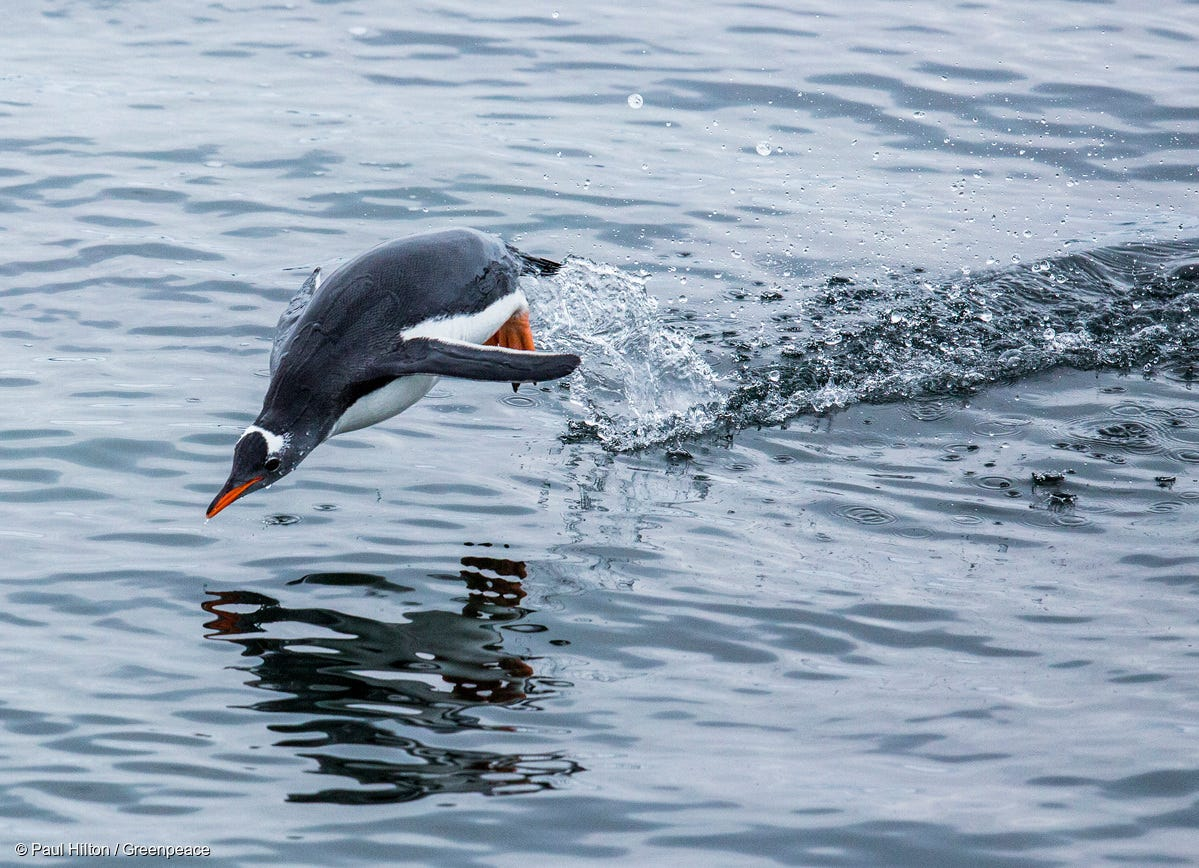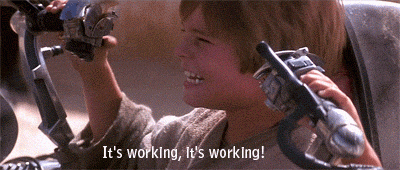# PenguinsPhoto Credit: @GreenpeaceUK on Medium

# Why?

This dataset in the package, {palmerpenguins}, is a great alternative to popular introductory data science datasets. One of the most popular datasets, isis, is beening phased out by the data science community due to the dataset’s author being a eugenicist. Although he made many great contributions to statistics, many in the data science community (and now the United States at large) condemn eugenics.

The palmerspenguins dataset is essentially the same idea as Fisher’s iris dataset in that they have the same potential for learning. These datasets are composed of 3 classes, that is, 3 different flowers or 3 different penguins). Each datapoint contains a series of independent variables, primarily measurements, which can be used to classify the data. For this new penguins dataset, there are variables:

• species

• island

• bill_length_mm

• bill_depth_mm

• flipper_length_mm

• body_mass_g

• sex

• year

and $$N=344$$.

# Data Visualization

What I really liked in this dataset was the bill_length_mm and bill_depth_mm; these measurements got me thinking it would be cool to plot an approximate beak profile for each penguin!

To do this I am going to construct a parabola for a crude approximation of beak profiles. I want a parabola that is rotated with its vertex to the right and the open side facing left… so a parabola in the family of $$x=y^2$$. To make this in R, I need to add some parameters and then make two curves, one for the top and one for the bottom ($$y=\pm \sqrt x$$). So I end up making parabolas of the form:

$y = \pm \sqrt (\frac{a-x}{b})$

So to make these curves, we will disctritize the range of penguin bills and compute the curve for each penguin given its bill measurements. First I need to make the function, then we will try a single penguin bill, then put them all together!

Below is the code that I used to construct a parabola for a beak. I break the function into a plus and a minus component and then return those vectors in a list object. I use parameters a as the bill length and b as bill depth. Bill depth must be halved to get the correct depth (making it depth instead of depth/2 would result in a doubly deep bill)!

beak_function <- function(x,a,b){
b <- b / 2

y1 <- ((a - x) / b) ^ 0.5
y2 <- (-((a - x) / b) ^ 0.5)

return(list(y1, y2))
}

So now that the functions is built, I can test it out on a sample penguin. I will use the first observation. So I take the list object and transform it into a dataframe, because {ggplot2}, and structure it with columns x, y1, y2. Time to see how it works!

param1 <- penguins$bill_length_mm param2 <- penguins$bill_depth_mm

test<-beak_function(seq(1,50,0.1),param1,param2)

test_df <-data.frame(x = seq(1,50,0.1),
y1 = test[],
y2 = test[])

test_df %>%
ggplot() +
geom_area(aes(x,y1), color = "orange", fill = "orange", alpha = 0.25)+
geom_area(aes(x,y2), color = "orange", fill = "orange", alpha = 0.25) +
geom_hline(yintercept = 0, color = "black")+
labs(title = "Penguin 1 Beak",
y = "Beak Depth (mm)",
x = "Bill Length (mm)") +
theme_light()
## Warning: Removed 109 rows containing missing values (position_stack).

## Warning: Removed 109 rows containing missing values (position_stack).Ha! It worked. Looks like a penguin beak to me. Amazing what we can do with {ggplot2}.Now, we do all the penguins. To visualize them all I want to facet by species, preserve the scale so we can compare across species, and make all of the curves fairly transparent so we can see them all together. Now, I made that plot above with a data frame, I need to do some data reformatting.

# Beak plotting params.
param1 <- penguins$bill_length_mm param2 <- penguins$bill_depth_mm
my_domain <- seq(1,65,0.1)

# Construct df and allocate storage.
beaks_df <- matrix(rep(0,(length(my_domain)*2*nrow(penguins))),
nrow = length(my_domain)*2)

# Loop each penguin, put in df.
for(i in 1:nrow(penguins)){
penguin_i <- beak_function(my_domain,param1[i],param2[i])

penguin_i_df <- data.frame(x = my_domain,
y1 = penguin_i[],
y2 = penguin_i[])

beaks_df[,i] <- c(penguin_i[],penguin_i[])
}

# Reformat df.
beaks_df <- beaks_df %>%
as.data.frame()

beaks_df$series <- c(rep("y1",length(my_domain)), rep("y2",length(my_domain))) beaks_df$x <- rep(my_domain,2)
colnames(beaks_df) <- c(paste0("p",seq(1,344)),"series","x")

beaks_plot <- beaks_df %>%
pivot_longer(cols = p1:p344)

penguins\$name <- paste0("p",seq(1,344))

beaks_plot <- left_join(beaks_plot, penguins, by = c("name"))
beaks_plot %>%
#filter(species == "Adelie", island == "Torgersen") %>%
arrange(desc(x)) %>%
mutate(super_group = paste(series,name)) %>%
group_by(super_group) %>%
ggplot()+
geom_line(aes(x = x, y = value, group = super_group), alpha = 0.5, color = "orange")+
geom_hline(yintercept = 0)+
facet_wrap(~species, nrow = 3)+
theme_light() + theme(aspect.ratio=0.25) +
labs(title = "Levi's Crude Penguin Beaks",
x = "Beak Length (mm)",
y = "Beak Depth (mm)")
## Warning: Removed 146932 row(s) containing missing values (geom_path).See the resemblence?

Great! This is what I wanted. I mean sure we could look at another plot, or summary statistics… but this is more fun. It looks like Gentoo have deeper beaks than Chinstrap, but only slightly. Adelie have the shortest beaks for sure! So now that we did that excessively difficult plot, we can look at the simpler plot that shows this same information better.

penguins %>%
ggplot(aes(x = bill_length_mm, y = bill_depth_mm, color = species))+
geom_point()+
scale_color_brewer(palette = "Set1")+
ggtitle("Better than Levi's First Plot")
## Warning: Removed 2 rows containing missing values (geom_point).This plot much better shows the differences in the species, and the few overlapping cases. You could make some easy decision boundaries on this plot! So although this plot is more appropriate… we made a fun one earlier!##### Levi C. Nicklas
###### Data Scientist

Graduate Student, Researcher, and Data Scientist.